Home > CC2MN > Chapter cc27 > Lesson cc27.2.2 > Problem7-112

7-112.
1. Solve the proportions using any strategy you choose. Show all of your steps. Homework Help ✎

1.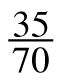=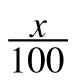2.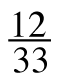=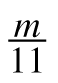3.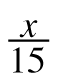=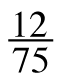4.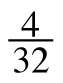=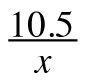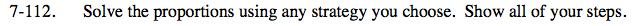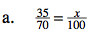Use cross multiplication.
35 · 100 = 70x
3500 = 70x

$x= \frac{3500}{70}=50$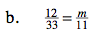Use the inverse to undo.

$\left(\frac{11}{1}\right)\frac{12}{33}=\frac{m}{11}\left(\frac{11}{1}\right)$

m = 4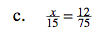Use a Giant One to find the equivalent ratio.

$\frac{x}{15}\left(\frac{?}{?}\right)=\frac{12}{75}$

$\frac{x}{15}\left(\frac{5}{5}\right)=\frac{12}{75}$

x = 2.4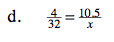Try using one of the methods presented in either part (a), (b), or (c).